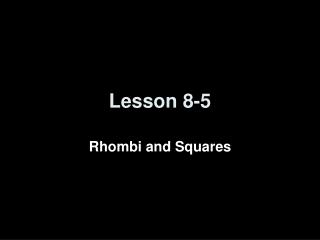Download PresentationLesson 8-5Lesson 8-5 - PowerPoint PPT Presentation

Download PresentationLesson 8-5
An Image/Link below is provided (as is) to download presentation

Download Policy: Content on the Website is provided to you AS IS for your information and personal use and may not be sold / licensed / shared on other websites without getting consent from its author. While downloading, if for some reason you are not able to download a presentation, the publisher may have deleted the file from their server.

- - - - - - - - - - - - - - - - - - - - - - - - - - - E N D - - - - - - - - - - - - - - - - - - - - - - - - - - -
Presentation Transcript

1. Lesson 8-5 Rhombi and Squares

2. Transparency 8-5 50 3 ± 4 52° 104° C 5-Minute Check on Lesson 8-4 WXYZ is a rectangle. Find each value. 1. If ZX = 6x – 4 and WY = 4x + 14, find ZX. 2. If WY = 26 and WR = 3y + 4, find y. 3. If mWXY = 6a² - 6, find a. RSTU is a rectangle. Find each value. 4. mVRS 5. mRVU 6. What are the coordinates of W if WXYZ is a rectangle and X(2,6), Y(4,3), and Z(1,1)? X W R Y Z S R V 38° U T Standardized Test Practice: (1,4) (1,-4) (-1,-4) (-1,4) A B C D Click the mouse button or press the Space Bar to display the answers.

3. Polygon Hierarchy Polygons Quadrilaterals Parallelograms Kites Trapezoids IsoscelesTrapezoids Rectangles Rhombi Squares

4. Objectives • Recognize and apply the properties of rhombi • All Parallelogram Properties • All 4 Sides Congruent • Diagonals bisect a pair of opposite ’s • Diagonals form right angles with each other • Recognize and apply the properties of squares • All Parallelogram Properties • All Rectangle Properties • All Rhombus Properties • Diagonals divide into 4 congruent ∆’s (45-45-90)

5. Vocabulary • Rhombus – quadrilateral with all four sides congruent • Square – a quadrilateral that is both a rhombus and a rectangle

6. Rhombi and Squares A B Rhombus CharacteristicsAll Parallelogram Properties All 4 Sides Congruent Diagonals bisect a pair of opposite ’s Diagonals form right angles with each other C D A B Square CharacteristicsAll Parallelogram PropertiesAll Rectangle Properties All Rhombus Properties Diagonals divide into 4 congruent ∆’s D C

7. N Example 5-2a Use rhombus LMNP to find the value of y if m1 = y² - 54. Diagonals of a rhombus are perpendicular. Substitution Add 54 to each side. Take the square root of each side. Answer: The value of y can be 12 or –12.

8. N Answer: Example 5-2c Use rhombus LMNP to find mPNL if mMLP = 64 Opposite angles are congruent. Substitution The diagonals of a rhombus bisect the angles.

9. a. b. Answer: Example 5-2e Use rhombus ABCD and the given information to find the value of each variable. Answer: 8 or –8

10. Example 5-4a A square table has four legs that are 2 feet apart. The table is placed over an umbrella stand so that the hole in the center of the table lines up with the hole in the stand. How far away from a leg is the center of the hole? Let ABCD be the square formed by the legs of the table. Since a square is a parallelogram, the diagonals bisect each other. Since the umbrella stand is placed so that its hole lines up with the hole in the table, the center of the umbrella pole is at point E, the point where the diagonals intersect. Use the Pythagorean Theorem to find the length of a diagonal.

11. The distance from the center of the pole to a leg is equal to the length of Example 5-4b Answer: The center of the pole is about 1.4 feet from a leg of a table.

12. Example 5-4d Kayla has a garden whose length and width are each 25 feet. If she places a fountain exactly in the center of the garden, how far is the center of the fountain from one of the corners of the garden? Answer: about 17.7 feet

13. Quadrilateral Characteristics Summary Convex Quadrilaterals 4 sided polygon 4 interior angles sum to 360 4 exterior angles sum to 360 Parallelograms Trapezoids Bases Parallel Legs are not Parallel Leg angles are supplementary Median is parallel to basesMedian = ½ (base + base) Opposite sides parallel and congruent Opposite angles congruent Consecutive angles supplementary Diagonals bisect each other Rectangles Rhombi IsoscelesTrapezoids All sides congruent Diagonals perpendicular Diagonals bisect opposite angles Angles all 90° Diagonals congruent Legs are congruent Base angle pairs congruent Diagonals are congruent Squares Diagonals divide into 4 congruent triangles

14. Summary & Homework • Summary: • A rhombus is a quadrilateral with each side congruent, diagonals that are perpendicular, and each diagonal bisecting a pair of opposite angles. • A quadrilateral that is both a rhombus and a rectangle is a square. • Homework: • pg 434-436; 14-23, 26-31